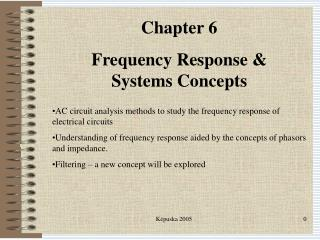Download PresentationChapter 6 Frequency Response & Systems Concepts

# Chapter 6 Frequency Response & Systems Concepts - PowerPoint PPT PresentationDownload Presentation## Chapter 6 Frequency Response & Systems Concepts

- - - - - - - - - - - - - - - - - - - - - - - - - - - E N D - - - - - - - - - - - - - - - - - - - - - - - - - - -
##### Presentation Transcript

1. Chapter 6 Frequency Response & Systems Concepts • AC circuit analysis methods to study the frequency response of electrical circuits • Understanding of frequency response aided by the concepts of phasors and impedance. • Filtering – a new concept will be explored Këpuska 2005

2. Objectives • Understand significance of frequency domain analysis • Introduction of Fourier series as a tool for computation of Fourier spectrum. • Analyze first and second-order electrical filters by determining their filtering properties. • Computation of frequency response and its graphical representation as Bode plot. Këpuska 2005

3. Sinusoidal Frequency Response • Provides a circuit response to a sinusoidal input of arbitrary frequency. • The frequency response of a circuit is a measure of voltage or current (magnitude and phase) as a function of the frequency of excitation (source) signal. Këpuska 2005

4. Methods to Compute Frequency Response • Thevenin equivalent source circuit: + Zs Z1 ZT VL ~ ~ VT VT Z2 - Këpuska 2005

5. Load Voltage VT ZT ZL ~ VT Këpuska 2005

6. Frequency Response • From definition: • VL(j) is a phase-shifted and amplitude-scaled version of VS(j) ⇨ Këpuska 2005

7. Frequency Response (cont) • Phasor form of the load voltage: Këpuska 2005

8. Example 6.1 • Compute the frequency response Hv(j) of the circuit for R1= 1k, C=10F; and RL= 10k. Këpuska 2005

9. Magnitude & Phaze Këpuska 2005

10. Fourier Analysis • Let x(t) be a periodic signal with period T. • x(t) = x(t+nT) for n=1,2,3,… Këpuska 2005

11. Fourier Series • A signal x(t) can be expressed as an infinite summation of sinusoidal components know as Fourier Series: • Sine-cosine (quadrature) representation • Magnitude and Phase form: • Fundamental Frequency and Period T: Këpuska 2005

12. Fourier Series • It can be shown • Or similarly Këpuska 2005

13. Fourier Series Aproximation • Infinite summation practically not possible • Replaced by finite summation that leads to approximation. • Higher order coefficients; n, are associated with higher frequencies; (2/T)n. ⇒ • Better approximations require larger bandwidths. Këpuska 2005

14. Odd and Even Functions Fourier Series Këpuska 2005

15. Frequency Spectrum Këpuska 2005

16. Computation of Fourier Series Coefficients Këpuska 2005

17. Example of Fourier Series Approximation • Square wave and its representation by a Fourier series. (a) Square wave (even function); (b) first three terms; (c) sum of first three terms Këpuska 2005

18. Example 6.3 Computation of Fourier Series Coefficients • Problem: Compute the complete Fourier Spectrum of the sawtooth function shown in the Figure below for T=1 and A=1: Këpuska 2005

19. Solution • x(t) is an odd function. • Evaluate the integral in equation Këpuska 2005

20. Solution (cont) • Spectrum computation: Këpuska 2005

21. Matlab Simulation • Components of the sawtooth wave function: Këpuska 2005

22. Matlab Simulation • Fourier Series approximation of sawtooth wave function Këpuska 2005

23. Example 6.4 • Problem: Compute the complete Fourier series expansion of the pulse waveform shown in the Figure for /T=0.2 • Plot the spectrum of the signal Këpuska 2005

24. Solution • Expression for x(t) • Evaluate Integral Equations: Këpuska 2005

25. Solution (cont) Këpuska 2005

26. Spectrum Computation • Magnitude: • Phase: Këpuska 2005

27. Graphical Representation Këpuska 2005

28. Matlab Simulation Këpuska 2005

29. Matlab Simulation Këpuska 2005

30. Linear Systems Response to Periodic Inputs • Any periodic signal x(t) can be represented as a sum of finite number of pure periodic terms: Këpuska 2005

31. General Input-Output Representation of a System Këpuska 2005

32. Linear Systems • For Linear Systems - by definition Principle of superposition applies: T{ax1(t) + bx2(t)} = aT{x1(t)} + bT{x2(t)} a x1 ax1[n] + bx2[n] T{} y= T{ax1(t) + bx2(t)} x2 b a aT{x1[n]} T{} x1 y= aT{x1(n)}+bT{x2(n)} T{} x2 bT{x2[n]} b Këpuska 2005

33. Linear System View of a Circuit • Output of a circuit y(t) as a function of the input x(t): Këpuska 2005

34. Example 6.6 Response of Linear System to Periodic Input • Problem: • Linear system: • Input: sawtooth waveform approximated with only first two Fourier components of the input waveform. Këpuska 2005

35. Solution • Approximation of the sawtooth function with first two terms of Fourier Series: • Spectrum Computation: Këpuska 2005

36. Frequency Response • Magnitude and Phase • Computation of Frequency Response for two frequency values of 1 = 8 and 2 = 16: Këpuska 2005

37. Frequency Response (cont) • Computation of steady-state periodic output of the system: Këpuska 2005

38. Matlab Simulation Këpuska 2005

39. Matlab Simulation Këpuska 2005

40. Filters • Low-Pass Filters Simple RC Filter Këpuska 2005

41. Low-Pass Filter Këpuska 2005

42. Low-Pass Filter • =0 • H(j)=1 ⇨ Vo(j)=Vi(j) • >0 Këpuska 2005

43. Low-Pass Filter Cutoff Frequency Këpuska 2005

44. Example 6.7 • Compute the response of the RC filter to sinusoidal inputs at the frequencies of 60 and 10,000 Hz. • R=1k, C=0.47F, vi(t)=5cos(t) V • 0=1/RC=2,128 rad/sec •  = 120 rad/sec ⇒ /0 = 0.177 •  = 20,000 rad/sec ⇒ /0 = 29.5 Këpuska 2005

45. Solution Këpuska 2005

46. High-Pass Filters Këpuska 2005

47. High-Pass Filter • The expression in previous slide can be written in magnitude-and-phase form: Këpuska 2005

48. High-Pass Filter Response Këpuska 2005

49. Band-Pass Filters Këpuska 2005

50. Frequency Response of Band-Pass Filter Këpuska 2005